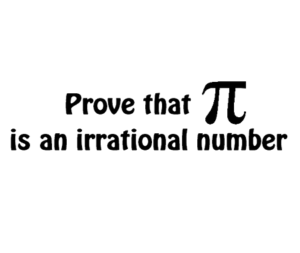Home -> Solved problems -> pi irrational number

## Is $$\pi$$ an irrational number ?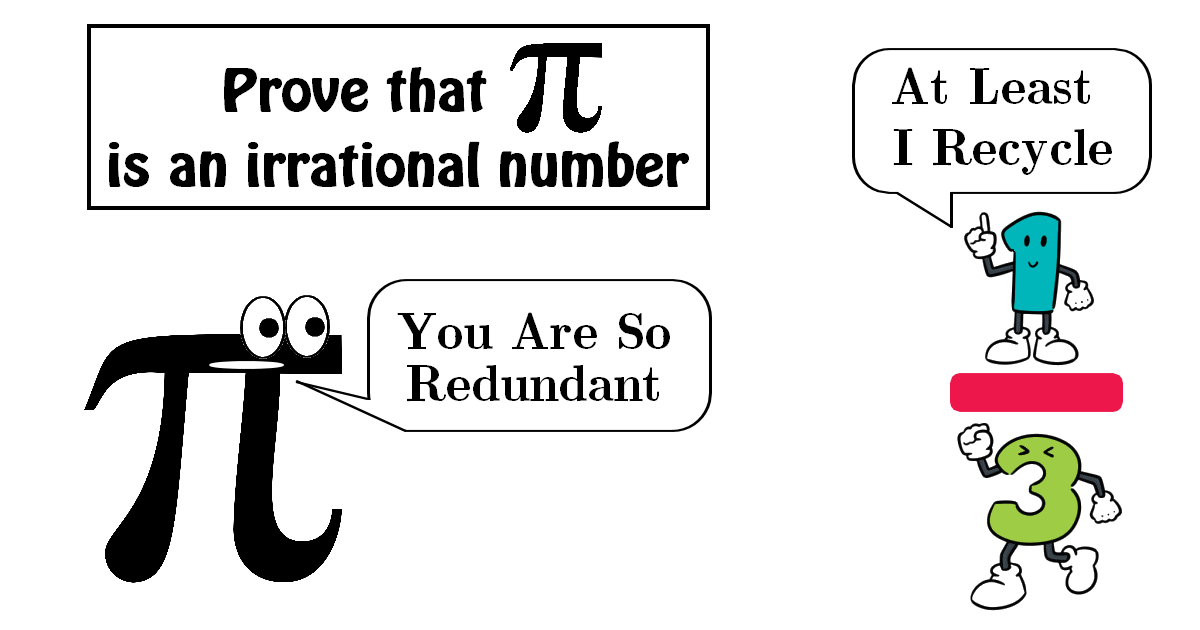### Solution

Let $$\pi=a/b$$, the quotient of two positive coprime integers. Let’s define the polynomials $f(x)=\frac{x^{n}(a-b x)^{n}}{n !}$ and $F(x)=f(x)-f^{(2)}(x)+f^{(4)}(x)-\cdots+(-1)^{n} f^{(2 n)}(x)$ We will specify the positive integer $$n$$ later. $$n ! f(x)$$ has integral coefficients and it is a function of $$x$$ of degree no fewer than $$n$$, $$f(x)$$ and its derivatives $$f^{(i)}(x)$$ have integral values for $$x=0$$ and also for $$x=\pi=\frac{a}{b}$$, since $$f(x)=f(\frac{a}{b}-x)$$. Considering elementary calculus we have
$\frac{d}{d x}\left\{F^{\prime}(x) \sin x-F(x) \cos x\right\}=F^{\prime \prime}(x) \sin x+F(x) \sin x=f(x) \sin x$ and $\int_{0}^{\pi} f(x) \sin x d x=\left[F^{\prime}(x) \sin x-F(x) \cos x\right]_{0}^{\pi}=F(\pi)+F(0)\;\;\;\;\;\;\;\text { (1) }$ We have $$F(\pi)+F(0)$$ is an integer, since $$f^{(i)}(\pi)$$ and $$f^{(i)}(0)$$ are integers. However for $$0<x<\pi$$, $0<f(x) \sin x<\frac{\pi^{n} a^{n}}{n !}$ The integral in $$\text { (1) }$$ is positive, but arbitrarily small for sufficiently large $$n$$ . Therefore $$\text { (1) }$$ is false, consequently $\large \pi \text { is an irrational number }$
Home -> Solved problems -> pi irrational number

### Related Topics

Find the volume of the square pyramid as a function of $$a$$ and $$H$$ by slicing method.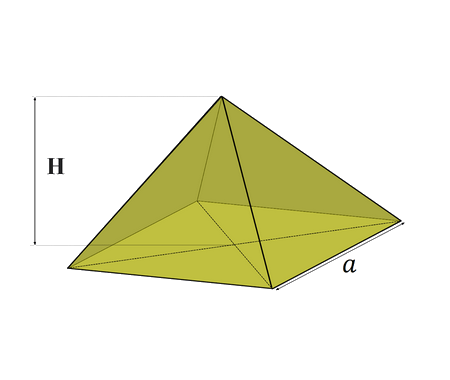Prove that $\lim_{x \rightarrow 0}\frac{\sin x}{x}=1$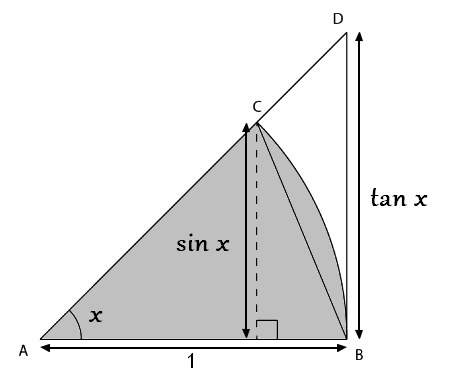Prove that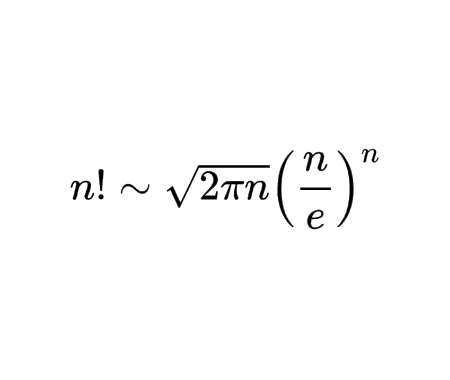Calculate the half derivative of $$x$$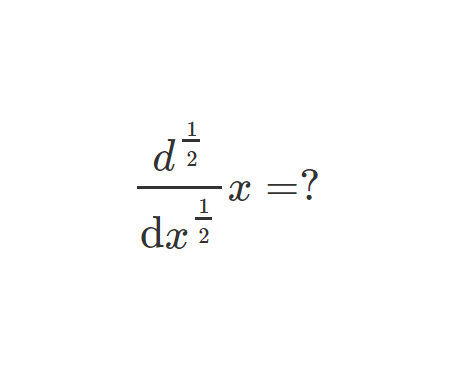Prove Wallis Product Using Integration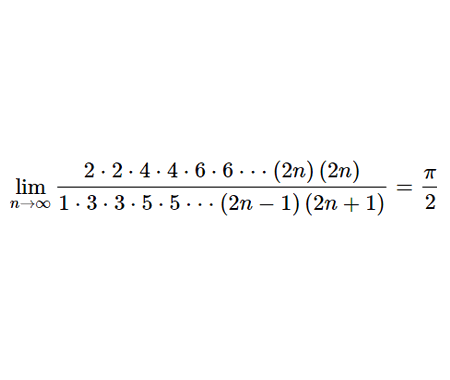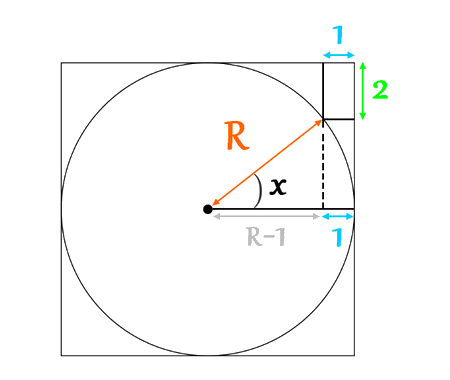Calculate the volume of Torus using cylindrical shells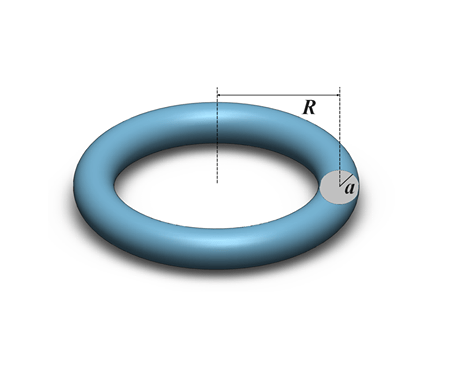Find the derivative of exponential $$x$$ from first principles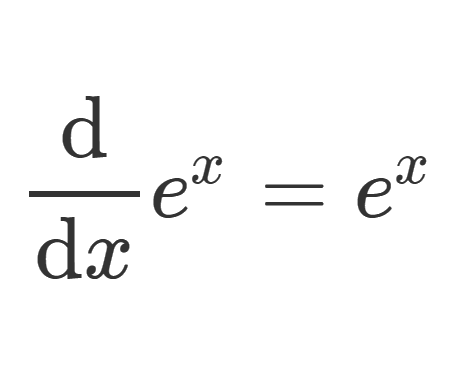Calculate the sum of areas of the three squares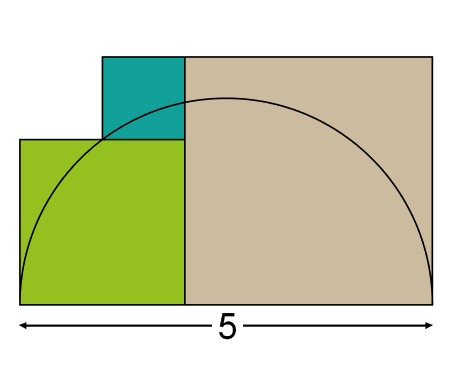Find the equation of the curve formed by a cable suspended between two points at the same height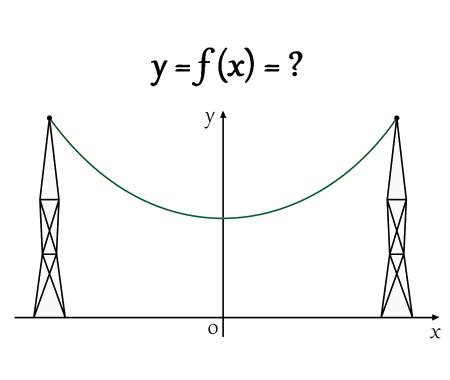Solve the equation for real values of $$x$$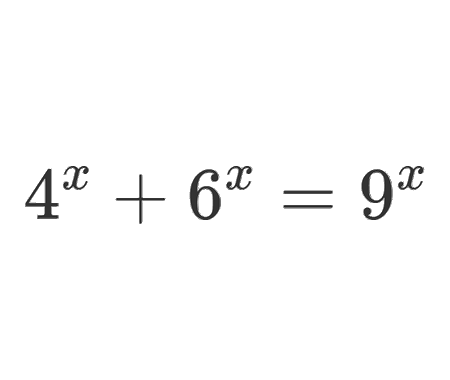Solve the equation for $$x\epsilon\mathbb{R}$$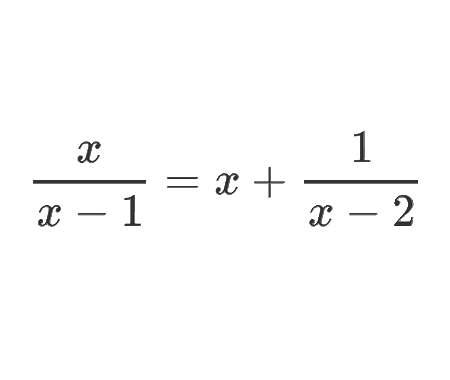Determine the angle $$x$$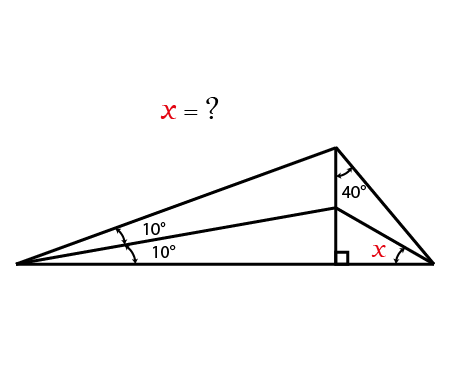Calculate the following limit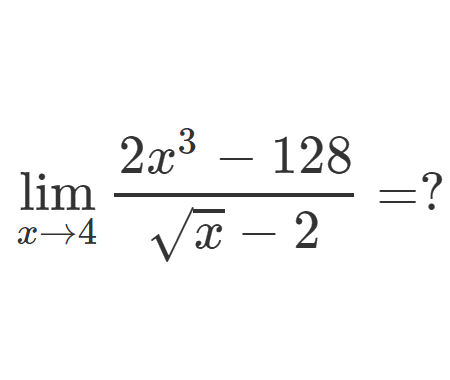Calculate the following limit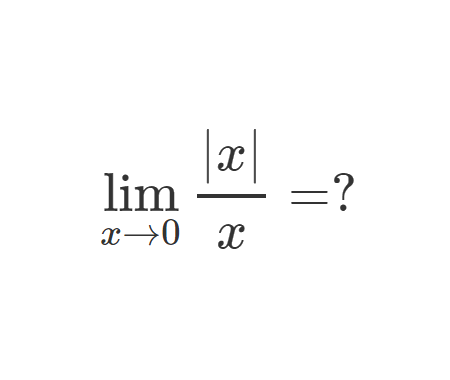Calculate the integral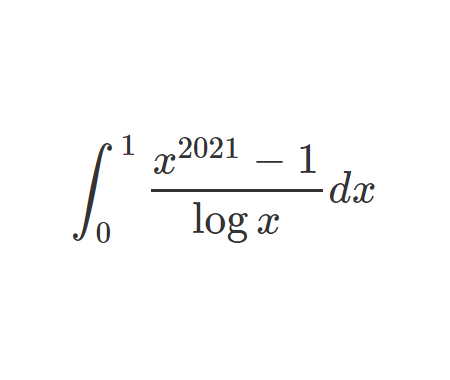Challenging problem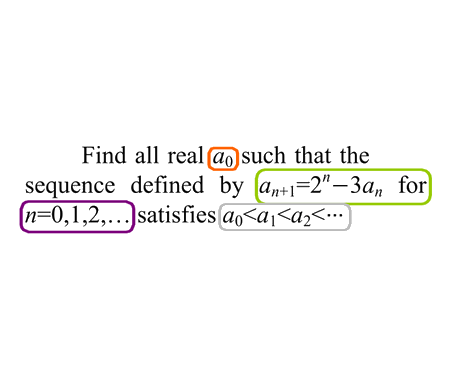Prove that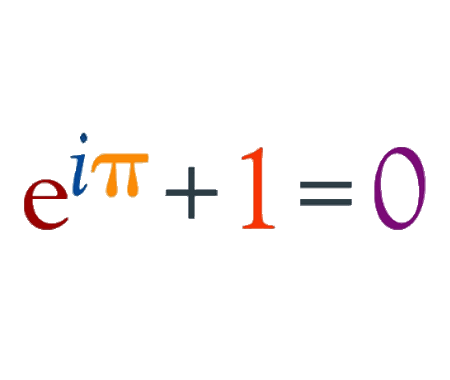Prove that $$e$$ is an irrational numberFind the derivative of $$y$$ with respect to $$x$$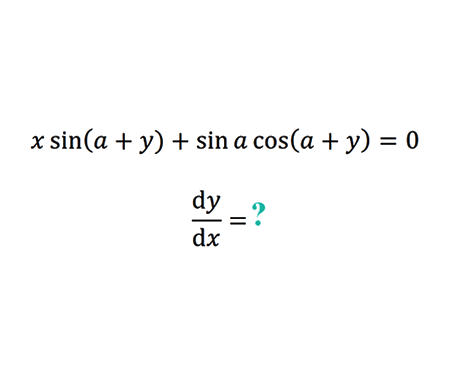Find the limit of width and height ratio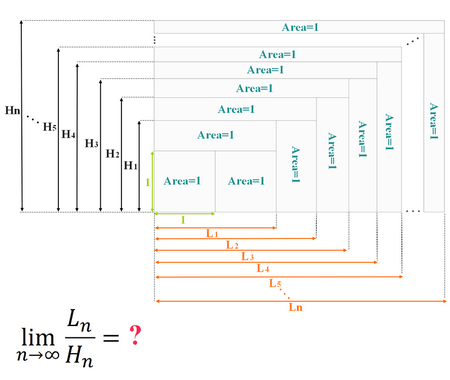How Tall Is The Table ?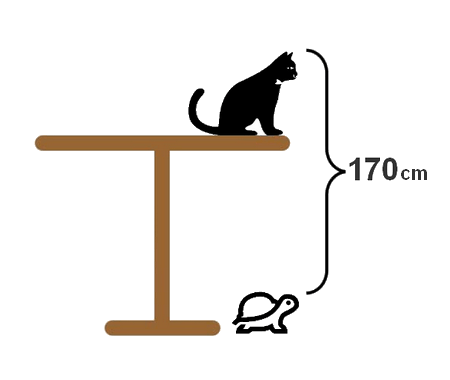Why 0.9999999...=1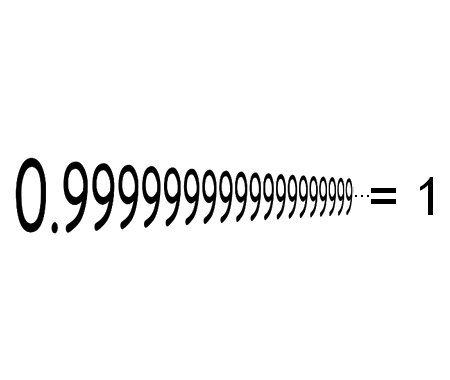Solve the equation for $$x \in \mathbb{R}$$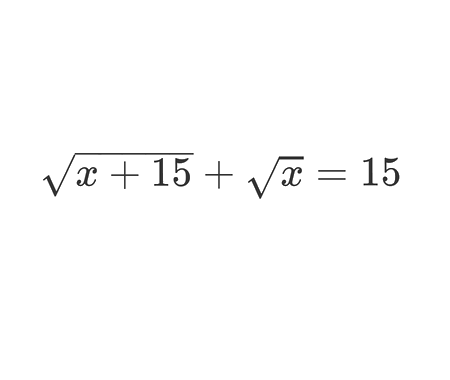Calculate the following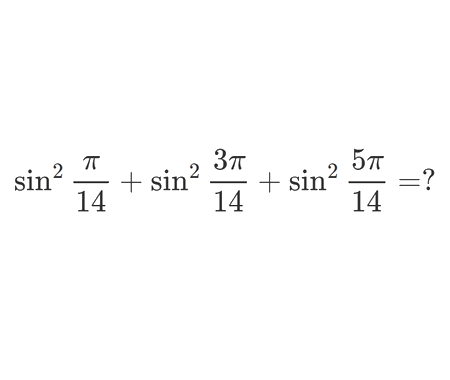Is $$\pi$$ an irrational number ?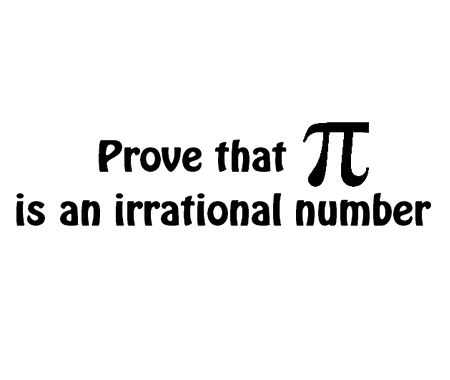Home -> Solved problems -> pi irrational number

#### Share the solution: pi irrational number